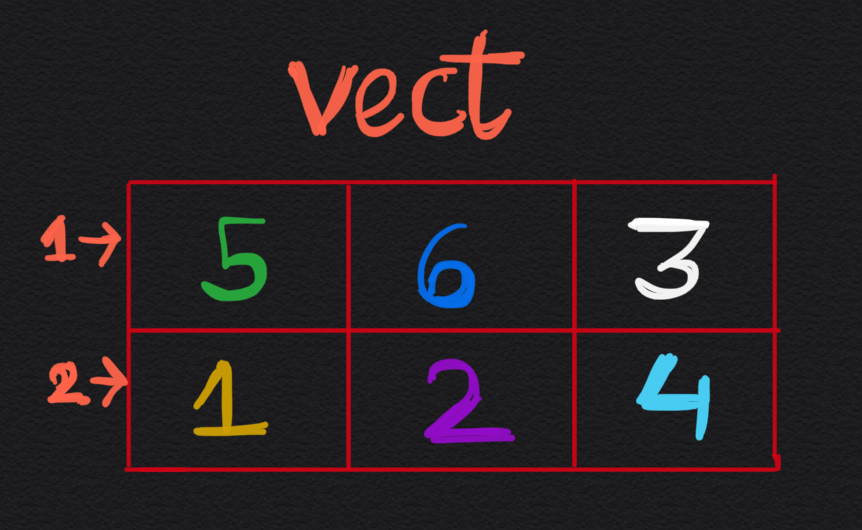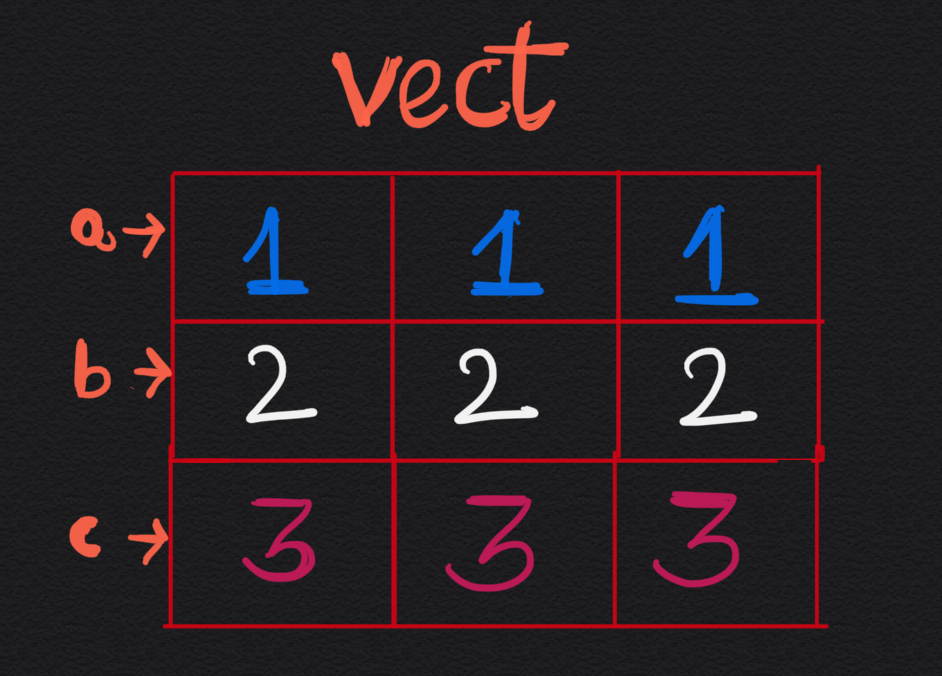×

Search anything:

# Defining a 2D vector in C++

#### Software Engineering C++Get this book -> Problems on Array: For Interviews and Competitive Programming

Reading time: 30 minutes | Coding time: 10 minutes

In this article, we have explored the different ways of defining a 2D vector in C++.

Before getting in the nitty-gritty of 2-D vectors, let us first define some working definitions of basic terms:

• Containers - These can be understood as implementation of abstract data types and as such are data structures available for use. e.g. arrays, set, map etc.
• Vectors - Vectors are containers which implement dynamic arrays or arrays which can change size according to our needs.

# A 2-D vector is a vector of vectors.

For e.g. you can define a two-dimensional vector of type T as follows:

``````std::vector<std::vector<T>> vector_name;
``````

Note: All the examples given ahead are for type int but they can easily be replicated for other types such as double, char etc. just by changing int in declaration to the required type.

Note:You can create a 2D array by doing int a, but std::vector<int> a(4,3) won't create a 2D vector - it will create a 4-element vector full of 3s.

# Syntax of defining 2-D vector

Initializing at the time of declaration:

``````std::vector<std::vector<int>> vect{{ 5, 6, 3 }, { 1, 2, 4 }};
``````Note: Languages like Java and C# allow for jagged arrays or 2-D arrays with variable columns in each row. In C++, we can achieve this by using 2-D vector as follows:

``````std::vector<std::vector<int>> vect{{ 5, 7, 12 }, { 8, 5 }, { 7, 8, 9, 0 }};
``````# Initializing a 2-D vector with help of 1-D vector

``````std::vector<int> va(3, 1);    // vector a {1, 1, 1}
std::vector<int> vb(3, 2);    // vector b {2, 2, 2}
std::vector<int> vc(3, 3);    // vector c {3, 3, 3}

std::vector<std::vector<int>> vect {va, vb, vc};
``````# Initializing a 2-D vector of n rows and m columns

``````std::vector<std::vector<int>> vect( n , std::vector<int> (m));
``````

Note: You can initialize the above vector with value k in each cell by using the following code:

``````std::vector<std::vector<int>>vect( n , std::vector<int>(m,k));
``````

# Applications

The applications of 2-D vectors are same as 2-D matrices even more as it is more versatile as we can add and delete rows according to our needs. Some uses are:

• To represent images and manipulate them
• To represent any 2-D grid
• Used in programming techniques like Dynamic Programming

## Exercise Problem

#### Write a program that takes input the no. of rows and the no. of columns in each row and outputs a 2-D vector giving output as in the following examples:

``````Input:
No. of rows = 4
No. of columns in rows = 5 2 4 1

Output:
1 2 3 4 5
1 2
1 2 3 4
1
``````
``````Input:
No. of rows = 3
No. of columns in rows = 5 6 1

Output:
1 2 3 4 5
1 2 3 4 5 6
1
``````

## Explanation of Solution

To solve this problem, we need to form a 2-D vector whose rows contains natural numbers starting from 1 to the no. of columns in that row. Also as we know that a 2-D vector is a vector of vectors. So we must first make a 2-D vector (named matrix here) using the following line:
``````std::vector <std::vector<int>> matrix;
``````

Then we will push required rows in it by first forming vector representing each row one by one and then pushing them in the 2-D vector. In the final step we print the 2-D vector.

Note: This problem can be solved by various methods like an array whose each cell points to a linked list but here we are demonstrating 2-D vectors. You are free to experiment and be creative in coming up with various approaches.

## Solution

``````#include <iostream>
#include <vector>

int main(){
//inputting the no. of rows in required 2-D matrix
int rows;
std::cout<<"No. of rows = ";
std::cin>>rows;

std::vector<int> columns;
std::cout<<"No. of columns in rows = ";
int temp;

//inputting the no. of columns in (i+1)th row
//and storing it in a vector
for(int i=0;i<rows;++i){
std::cin>>temp;
columns.push_back(temp);
}

//defining the 2-D vector
std::vector <std::vector<int>> matrix;
for(int i=0;i<rows;++i){

//defining a temporary vector
std::vector <int> tempvect;
//filling temporary vector with required
//values for (i+1)th row from top
for(int j=0;j<columns[i];++j){
tempvect.push_back(j+1);
}

//saving the created row in matrix
matrix.push_back(tempvect);
}

//printing the matrix
for(int i=0;i<matrix.size();++i){
//printing the (i+1)th row(or vector) from top
for(int j=0;j<matrix[i].size();++j){
std::cout<<matrix[i][j]<<" ";
}
std::cout<<"\n";
}
return 0;
}
``````

# Time Complexity

• Worst case time complexity: `O(N)`
• Average case time complexity: `Θ(N)`
• Best case time complexity: `Ω(N)`
• Space complexity: `Θ(N)`

where N is the summation of all columns.

With this article at OpenGenus, you must have the complete idea of defining a 2D vector in C++. Enjoy.